# 第 7 章 数据可视化

library(tidyverse) # install.packages("tidyverse")
library(patchwork) # install.packages("patchwork")

## 7.1 为什么要可视化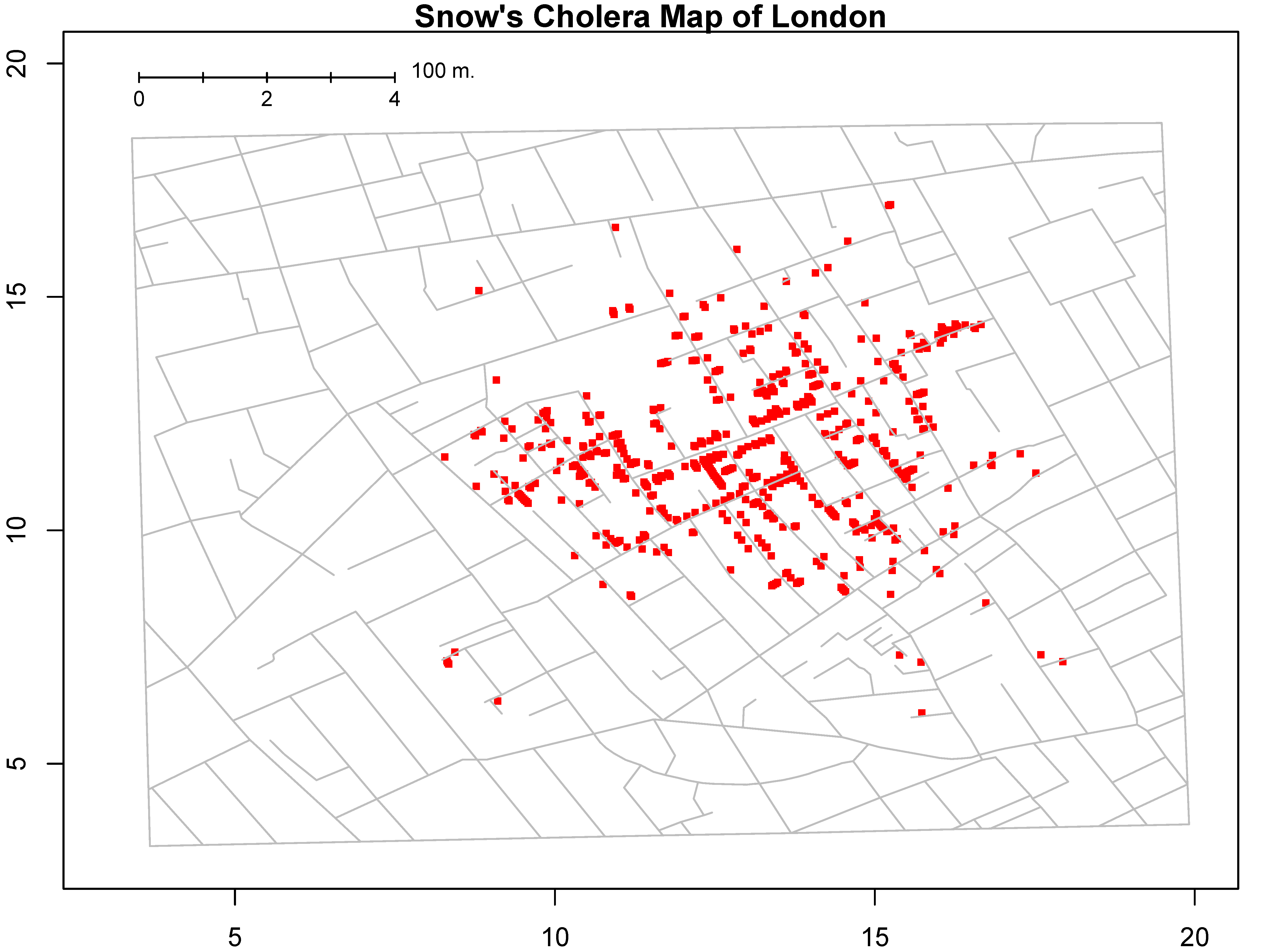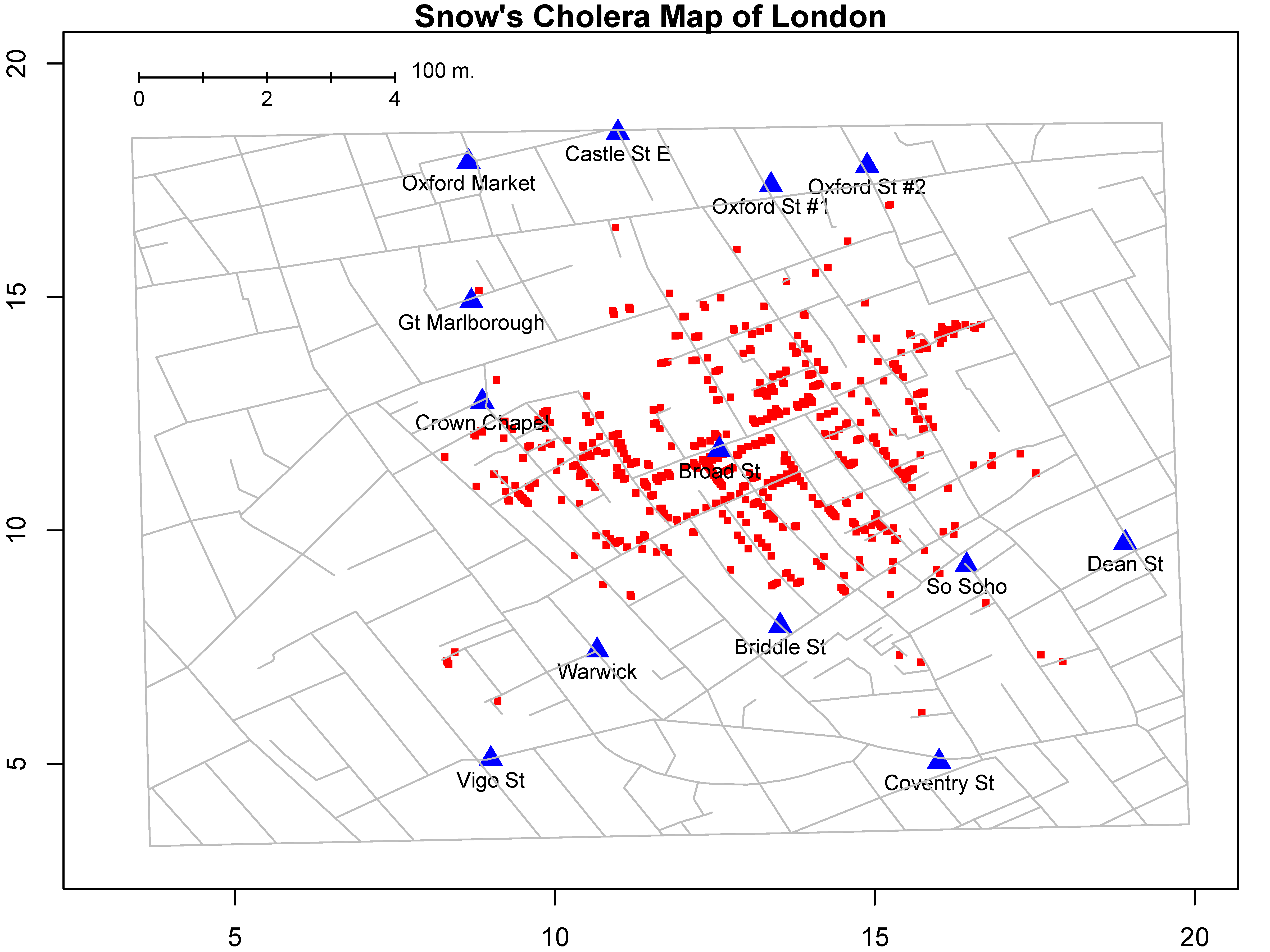## 7.2 什么是数据可视化

### 7.2.1 图形属性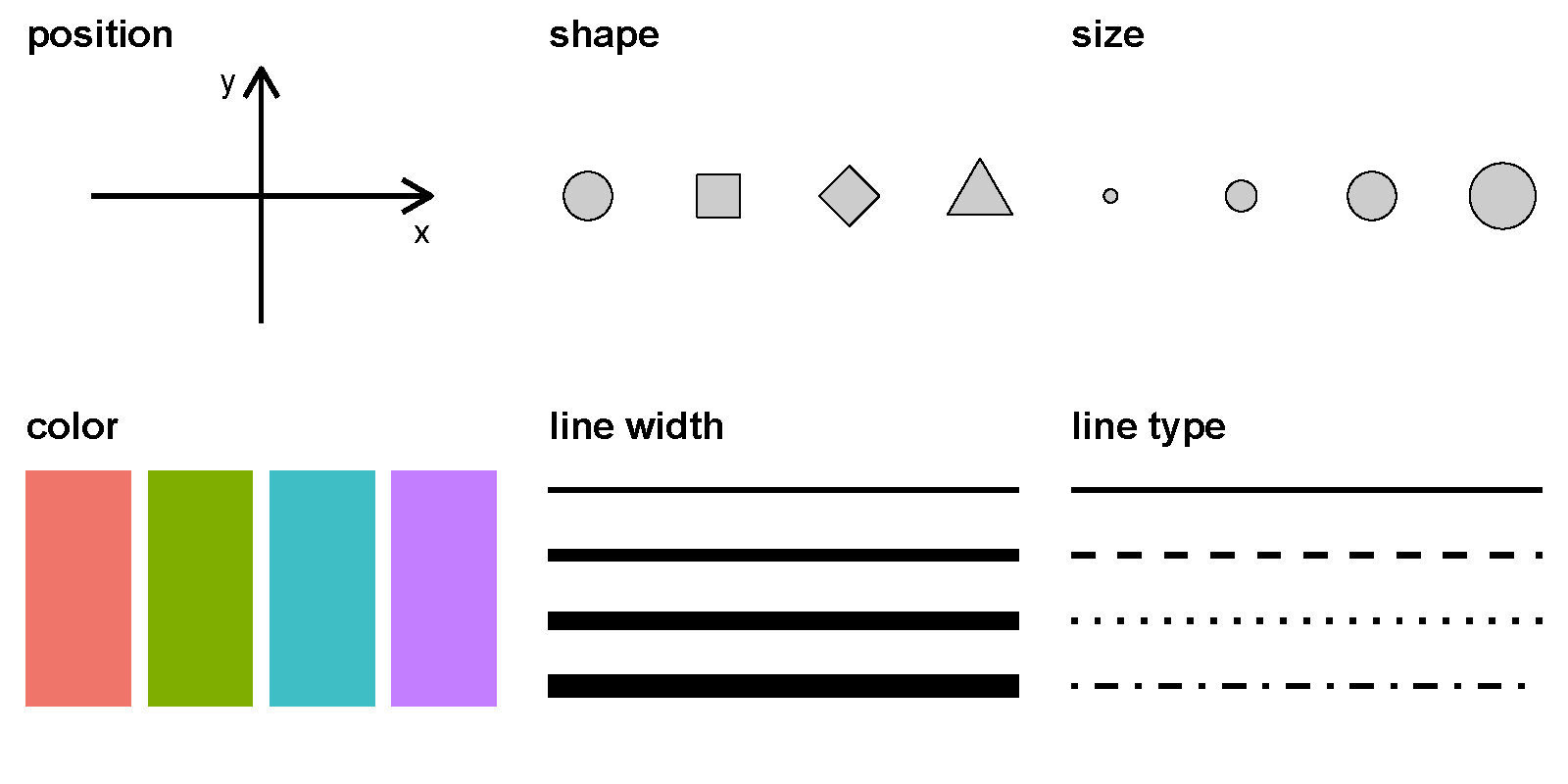## 7.3 宏包ggplot2

library(cranlogs)

sum(d\$count)
##  9889742

## 7.4 ggplot2 的图形语法

ggplot2有一套优雅的绘图语法，包名中“gg”是grammar of graphics的简称。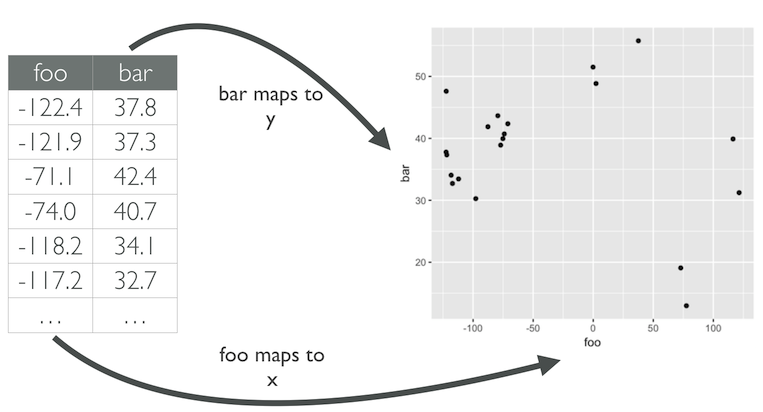ggplot()函数包括9个部件：

• 数据 (data) （ 数据框）
• 映射 (mapping)
• 几何对象 (geom)
• 统计变换 (stats)
• 标度 (scale)
• 坐标系 (coord)
• 分面 (facet)
• 主题 (theme)
• 存储和输出 (output)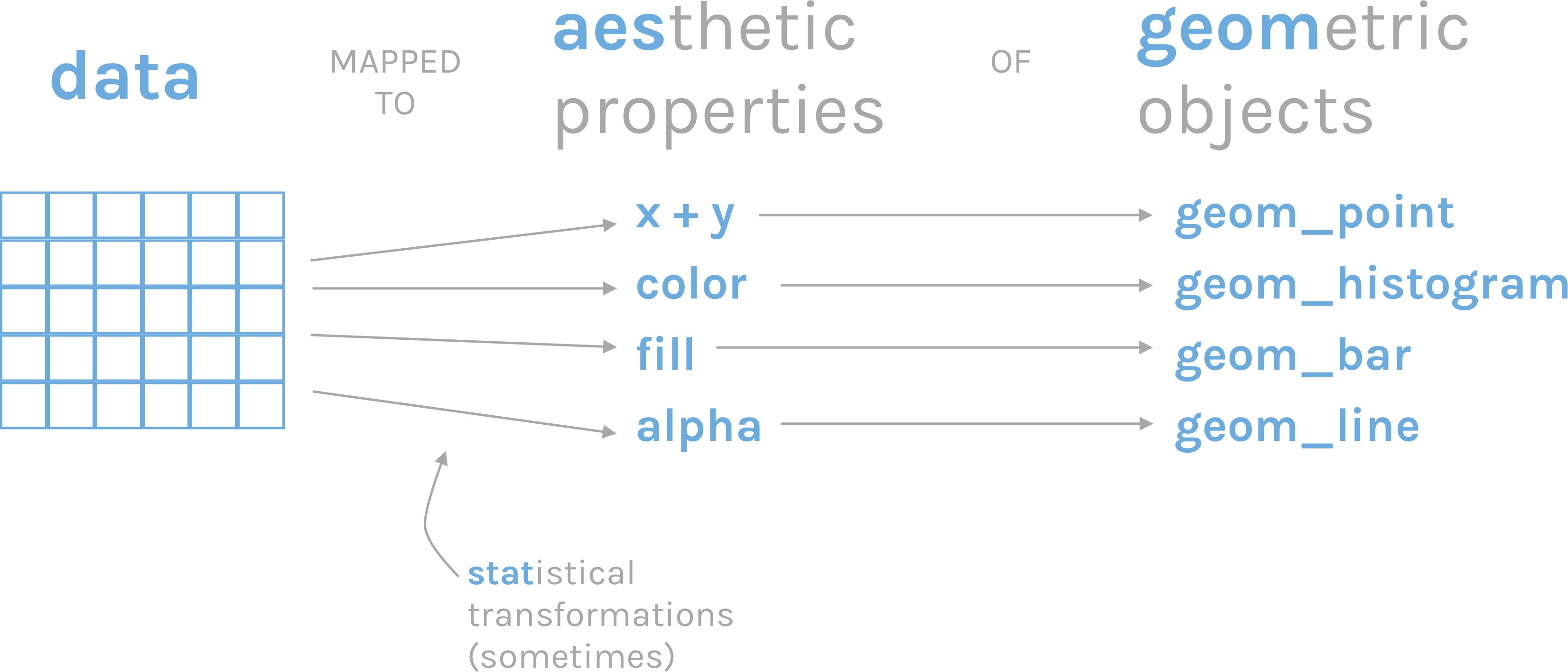### 7.4.1 语法模板library(tidyverse)
d
## # A tibble: 135 x 5
##     year temp_anomaly land_anomaly ocean_anomaly
##    <dbl>        <dbl>        <dbl>         <dbl>
##  1  1880        -0.11        -0.48         -0.01
##  2  1881        -0.08        -0.4           0.01
##  3  1882        -0.1         -0.48          0
##  4  1883        -0.18        -0.66         -0.04
##  5  1884        -0.26        -0.69         -0.14
##  6  1885        -0.25        -0.56         -0.17
##  7  1886        -0.24        -0.51         -0.17
##  8  1887        -0.28        -0.47         -0.23
##  9  1888        -0.13        -0.41         -0.05
## 10  1889        -0.09        -0.31         -0.02
## # ... with 125 more rows, and 1 more variable:
## #   carbon_emissions <dbl>
library(ggplot2)
ggplot(data = d, mapping = aes(x = year, y = carbon_emissions)) +
geom_line() +
xlab("Year") +
ylab("Carbon emissions (metric tons)") +
ggtitle("Annual global carbon emissions, 1880-2014")## 7.5 映射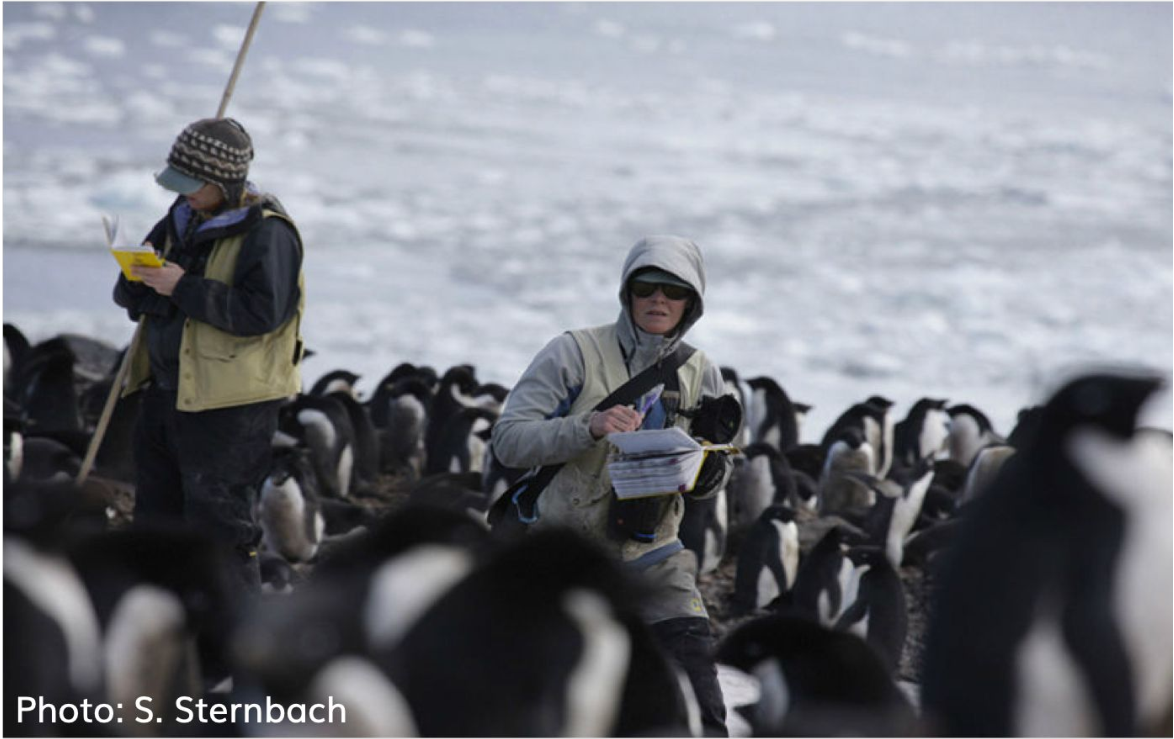library(tidyverse)
janitor::clean_names() %>%
drop_na()

penguins %>%
head()
## # A tibble: 6 x 8
##   species island bill_length_mm bill_depth_mm
##   <chr>   <chr>           <dbl>         <dbl>
## 1 Adelie  Torge~           39.1          18.7
## 2 Adelie  Torge~           39.5          17.4
## 3 Adelie  Torge~           40.3          18
## 4 Adelie  Torge~           36.7          19.3
## 5 Adelie  Torge~           39.3          20.6
## 6 Adelie  Torge~           38.9          17.8
## # ... with 4 more variables: flipper_length_mm <dbl>,
## #   body_mass_g <dbl>, sex <chr>, year <dbl>

### 7.5.1 变量含义

variable class description
species integer 企鹅种类 (Adelie, Gentoo, Chinstrap)
island integer 所在岛屿 (Biscoe, Dream, Torgersen)
bill_length_mm double 嘴峰长度 (单位毫米)
bill_depth_mm double 嘴峰深度 (单位毫米)
flipper_length_mm integer 鰭肢长度 (单位毫米)
body_mass_g integer 体重 (单位克)
sex integer 性别
year integer 记录年份

### 7.5.2 嘴巴越长，嘴巴也会越厚？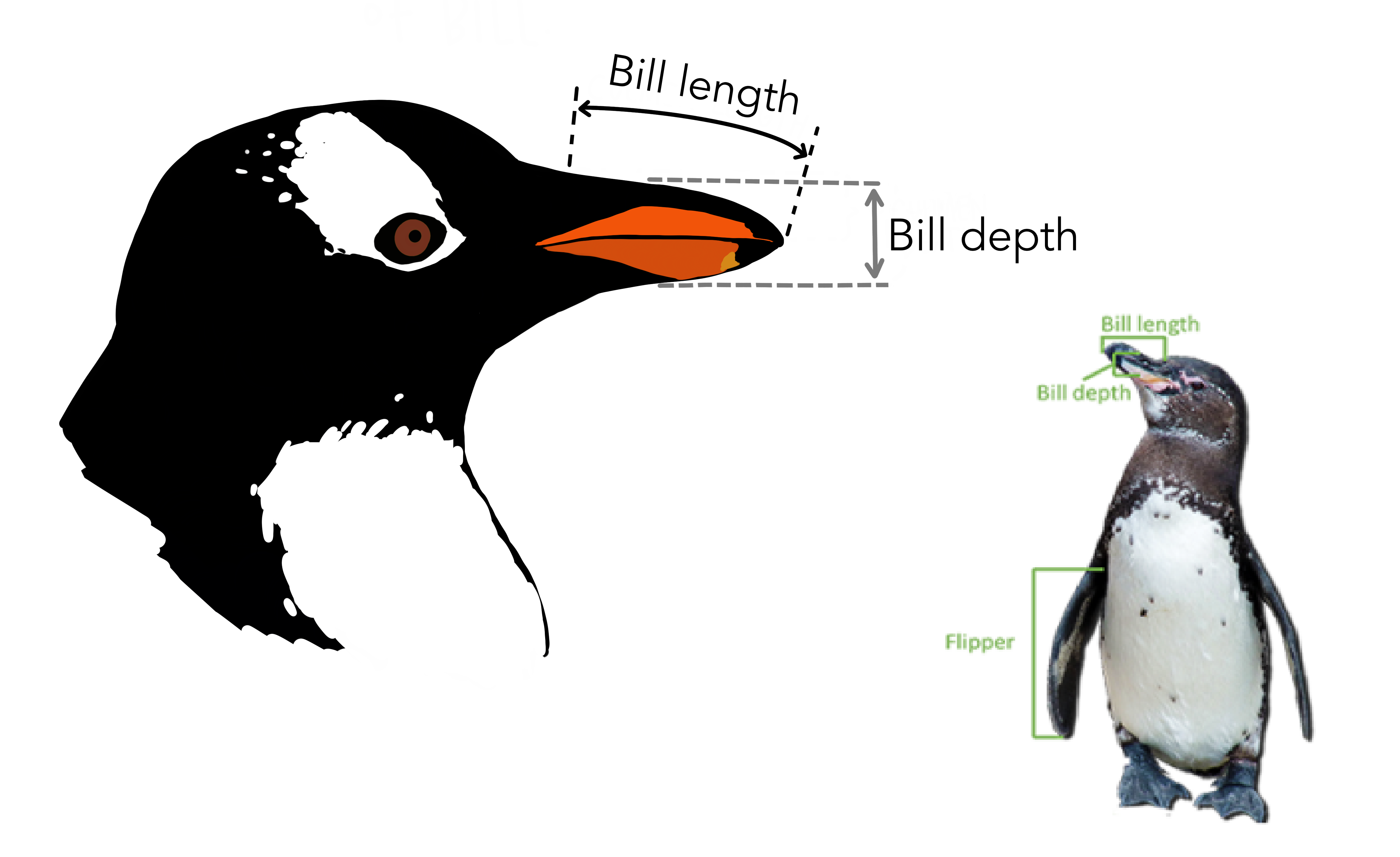penguins %>%
select(species, sex, bill_length_mm, bill_depth_mm) %>%
head(4)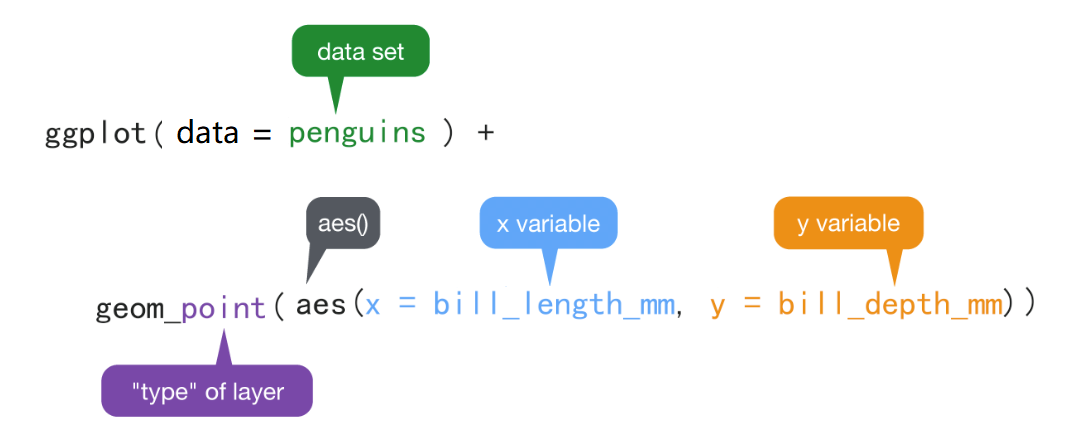• ggplot()表示调用该函数画图，data = penguins 表示使用penguins这个数据框来画图。

• aes()表示数值和视觉属性之间的映射。

aes(x = bill_length_mm, y = bill_depth_mm)，意思是变量bill_length_mm作为（映射为）x轴方向的位置，变量bill_depth_mm作为（映射为）y轴方向的位置

• aes()除了位置上映射，还可以实现色彩、形状或透明度等视觉属性的映射。

• geom_point()表示绘制散点图。

• +表示添加图层。ggplot(penguins,
aes(x = bill_length_mm, y = bill_depth_mm, color = species)) +
geom_point()ggplot(penguins,
aes(x = bill_length_mm, y = bill_depth_mm, size = species)) +
geom_point()
ggplot(penguins,
aes(x = bill_length_mm, y = bill_depth_mm, shape = species)) +
geom_point()
ggplot(penguins,
aes(x = bill_length_mm, y = bill_depth_mm, alpha = species)) +
geom_point()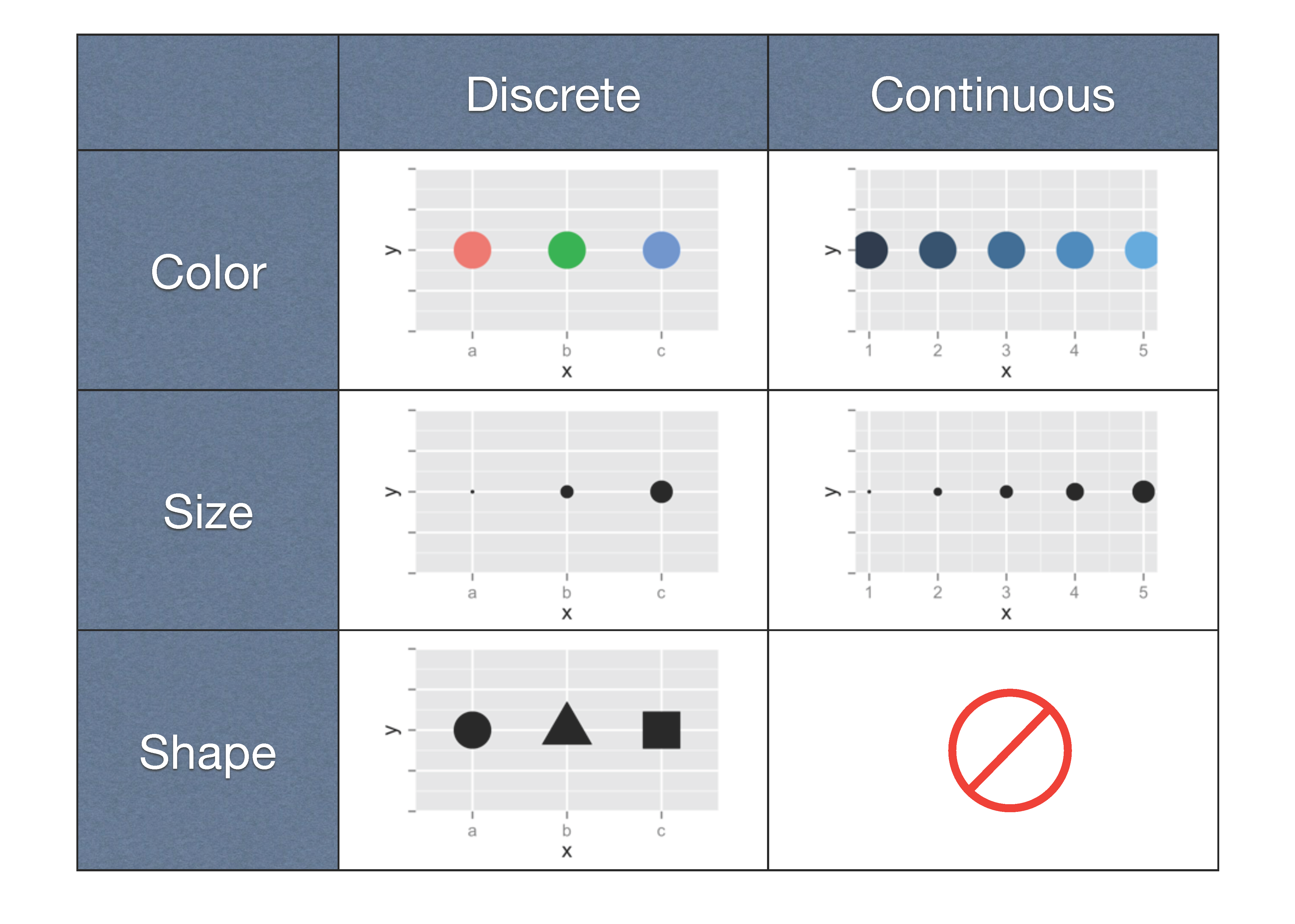## 7.6 映射 vs.设置

ggplot(penguins,
aes(x = bill_length_mm, y = bill_depth_mm)) +
geom_point(color = "blue")ggplot(penguins,
aes(x = bill_length_mm, y = bill_depth_mm)) +
geom_point(size = 5)
ggplot(penguins,
aes(x = bill_length_mm, y = bill_depth_mm)) +
geom_point(shape = 2)
ggplot(penguins,
aes(x = bill_length_mm, y = bill_depth_mm)) +
geom_point(alpha = 0.5)

### 7.6.1 提问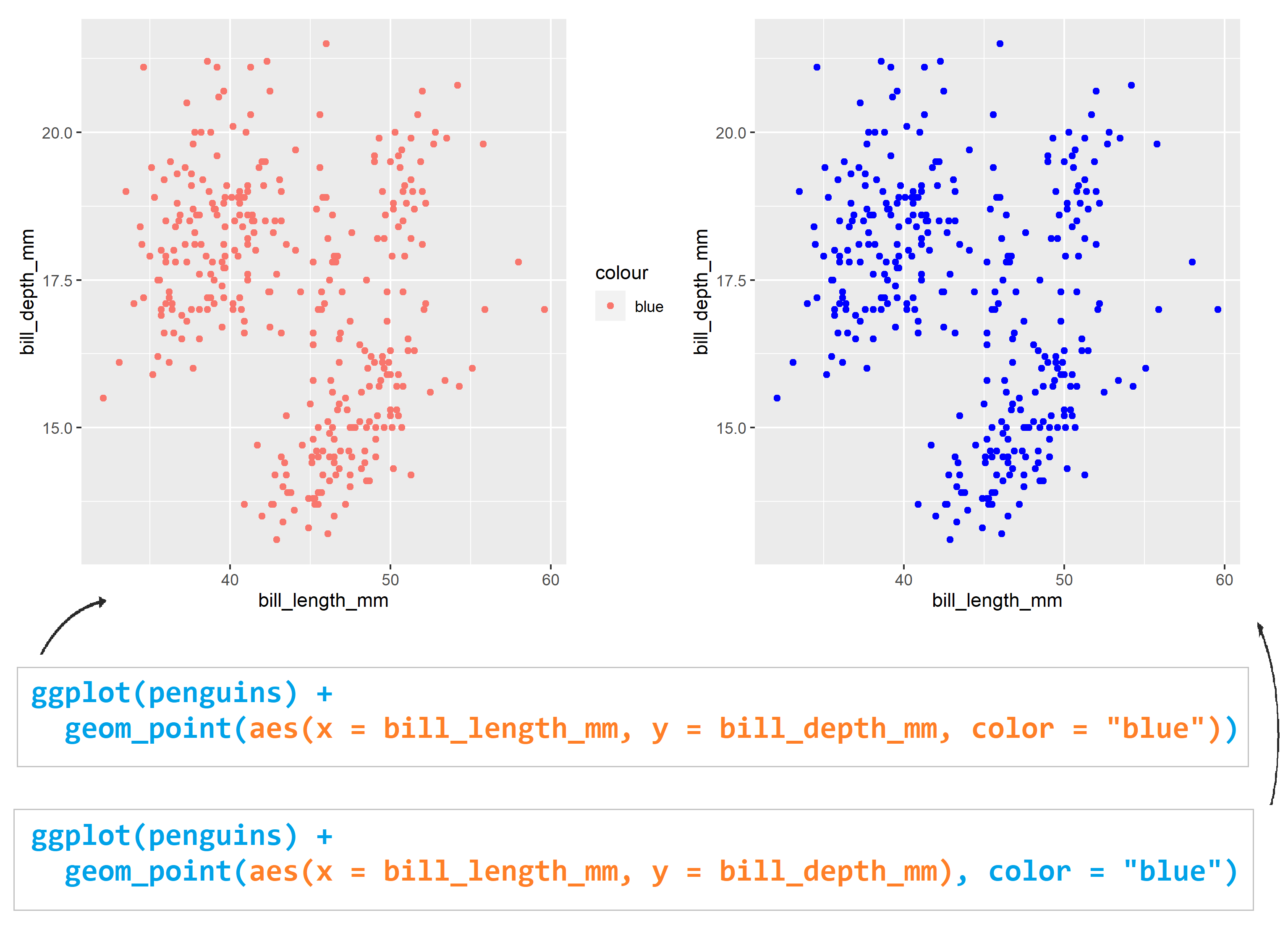## 7.7 几何对象

geom_point() 可以画散点图，也可以使用geom_smooth()绘制平滑曲线，

p1 <-
ggplot(penguins, aes(x = bill_length_mm, y = bill_depth_mm)) +
geom_point()
p1p2 <-
ggplot(penguins, aes(x = bill_length_mm, y = bill_depth_mm)) +
geom_smooth()
p2## 7.8 图层叠加

p3 <-
ggplot(penguins, aes(x = bill_length_mm, y = bill_depth_mm)) +
geom_point() +
geom_smooth()
p3library(patchwork)
(p1 / p2) | p3## 7.9 Global vs. Local

ggplot(penguins, aes(x = bill_length_mm, y = bill_depth_mm, color = species)) +
geom_point()ggplot(penguins) +
geom_point(aes(x = bill_length_mm, y = bill_depth_mm, color = species))ggplot(penguins, aes(x = bill_length_mm, y = bill_depth_mm, color = species)) +
geom_point()

ggplot(penguins) +
geom_point(aes(x = bill_length_mm, y = bill_depth_mm, color = species))

ggplot(penguins, aes(x = bill_length_mm, y = bill_depth_mm)) +
geom_point(aes(color = species)) +
geom_smooth()

ggplot(penguins,aes(x = bill_length_mm, y = bill_depth_mm, color = species)) +
geom_point(aes(color = sex))ggplot(penguins, aes(x = bill_length_mm, y = bill_depth_mm, color = species)) +
geom_smooth(method = lm) +
geom_point()ggplot(penguins, aes(x = bill_length_mm, y = bill_depth_mm)) +
geom_smooth(method = lm) +
geom_point(aes(color = species))## 7.10 保存图片

p <- penguins %>%
ggplot(aes(x = bill_length_mm, y = bill_depth_mm)) +
geom_smooth(method = lm) +
geom_point(aes(color = species)) +
ggtitle("This is my first plot")

ggsave(
filename = "myfirst_plot.pdf",
plot = p,
width = 8,
height = 6,
dpi = 300
)

## 7.11 课堂作业

• 企鹅嘴巴长度和嘴巴厚度的散点图
• 不同企鹅种类用不同的颜色
• 整体的线性拟合
• 不同种类分别线性拟合
ggplot(penguins, aes(x = ___, y = ___)) +
geom_point() +
geom_smooth() +
geom_smooth()